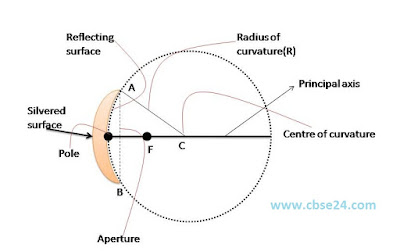# Basic of spherical mirror and relation between f and R class 10 | www.cbse24.com

### Reflection of light from curved surface: spherical mirrors

A spherical mirror is a reflecting surface which forms parts of a hollow sphereImage of concave and convex mirror

### Basic definition and connection with spherical concave mirror1:Pole:-It is the middle Point P of the the spherical mirror.

2: Center of curvature: It is the center C of the sphere of which the mirror forms a part

3:Radius of curvature: It is the radius(R=AB or BC) of the sphere of which the mirror form a part

4:Principal axis:-The line PC passing through the pole and boundary of the spherical mirror at its center of curvature c.

5:Linear aperture:-It is the diameter Ab of the circular boundary of the spherical mirror.

6:-Angular Aperture:-It is the angle ACB subtended by the boundary of the spherical mirror at its center of curvature C

7:-Principal focus:-A narrow beam of light parallel to the principal axis either actually converges to as appears to diverge from a point F on the principal axis after reflection from the spherical mirror. This point is called the principal focus of the mirror. A concave mirror has a real focus while a convex mirror has virtual focus

8:-Focal length:-It is distance(f=PF) between the focus and the pole of the mirror### Relation between f and R:f=R/2

or R=2f

*